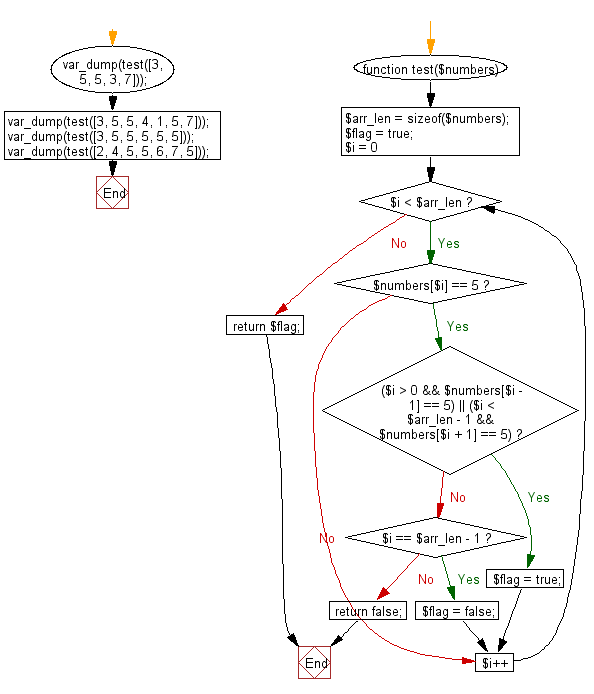﻿ PHP Exercises: Check a given array of integers and return true if every 5 that appears in the given array is next to another 5 - w3resource# PHP Exercises: Check a given array of integers and return true if every 5 that appears in the given array is next to another 5

## PHP Basic Algorithm: Exercise-121 with Solution

Write a PHP program to check a given array of integers and return true if every 5 that appears in the given array is next to another 5.

Sample Solution:

PHP Code :

``````<?php
function test(\$numbers)
{
\$arr_len = sizeof(\$numbers);
\$flag = true;

for (\$i = 0; \$i < \$arr_len; \$i++)
{
if (\$numbers[\$i] == 5)
{
if ((\$i > 0 && \$numbers[\$i - 1] == 5) || (\$i < \$arr_len - 1 && \$numbers[\$i + 1] == 5)) \$flag = true;
else if (\$i == \$arr_len - 1) \$flag = false;
else return false;
}
}
return \$flag;
}

var_dump(test([3, 5, 5, 3, 7]));
var_dump(test([3, 5, 5, 4, 1, 5, 7]));
var_dump(test([3, 5, 5, 5, 5, 5]));
var_dump(test([2, 4, 5, 5, 6, 7, 5]));
``````

Sample Output:

```bool(true)
bool(false)
bool(true)
bool(false)
```

Flowchart:PHP Code Editor:

What is the difficulty level of this exercise?

﻿

## PHP: Tips of the Day

\$_REQUEST: This SuperGlobal Variable is used to collect data submitted by a HTML Form

Example:

This code save in a php file and run in the browser.

```<!DOCTYPE html>
<html>
<body>

<form method="post" action="<?php echo \$_SERVER['PHP_SELF'];?>">
NAME: <input type="text" name="user">
<button type="submit">SUBMIT</button>
</form>
<?php
if (\$_SERVER["REQUEST_METHOD"] == "POST") {
\$name = htmlspecialchars(\$_REQUEST['user']);
if(empty(\$name)){
echo "Name is empty";
} else {
echo \$name;
}
}
?>
</body>
</html>
```

Output:

```Owen
```